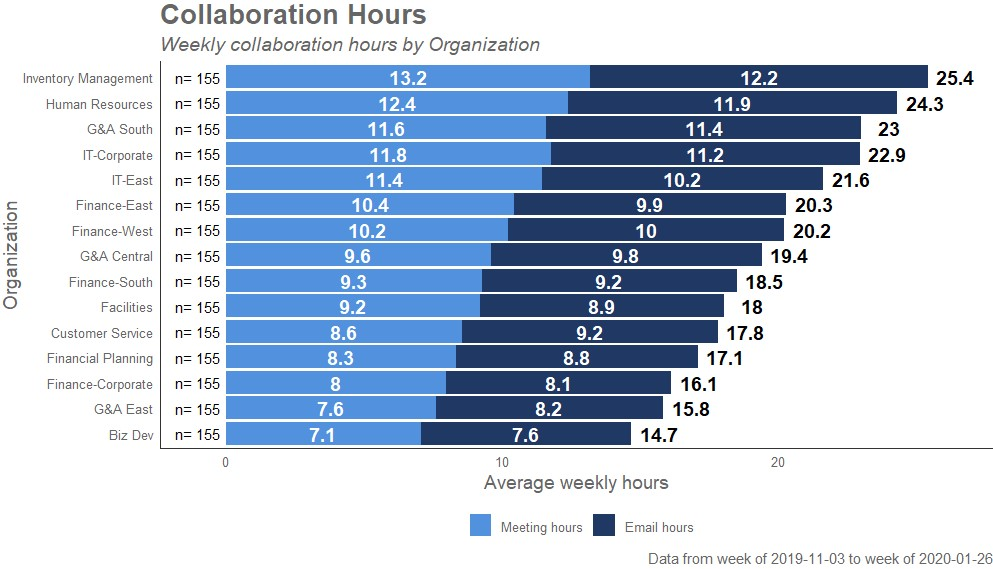There are four main types of functions in wpa: 1. Standard Analysis 2. Report Generation 3. Advanced / Support Functions 4. Sample datasets

### 1. Standard Analysis

Standard Analysis functions are the most common type of functions in wpa. They typically accept a data frame as an input (usually requiring a Standard Person Query), and can return either a pre-designed graph as a ggplot object, or a summary data table as a data frame.

Examples: - collaboration_dist() - meeting_summary() - email_trend() - collaboration_sum()

Here is an example of collaboration_sum():

collaboration_sum(sq_data, return = "plot")For the standard functions, there are six basic plot types which could be paired with six different key metrics. The six plot types are:

1. _summary(): produces a summary bar plot of the metric.
2. _dist(): produces a stacked bar plot of the metric.
3. _fizz(): produces a jittered, ‘fizzy drink’ plot of the metric.
4. _line(): produces a time-series line plot of the metric, with organizational attributes shown as facets.
5. _trend(): produces heatmap bars of the metric to show intensity over time.
6. _rank(): produces a rank table of all sub-groups (as per a set of organizational attributes) for a given metric. This is the only exception where the function returns a data frame by default, rather than a plot.

The six key metrics are:

1. collab: stands for Collaboration Hours, and uses the metric Collaboration_hours.
2. email: stands for Email Hours, and uses the metric Email_hours.
3. meeting: stands for Meeting Hours, and uses the metric Meeting_hours.
4. afterhours: stands for After-hours Collaboration Hours, and uses the metric After_hours_collaboration_hours.
5. one2one: stands for one-to-one collaboration hours with direct manager. Uses the metric Meeting_hours_with_manager_1_on_1.
6. workloads: stands for Work Week Span, and uses the metric Workweek_span.

You can combine the plot types and the key metrics (as prefixes and suffixes) to generate the desired output, e.g. email_ and dist for email_dist().

For more advanced users, there are also a number of flexible analysis functions which allow you to generate the plots with any Workplace Analytics metric, where the metric name needs to be supplied in addition to the function. For instance,

create_bar(sq_data, metric = "Email_hours")

would return a similar result as email_summary(sq_data), but where you can replace the metric with one of your own choice. Here are some of the available flexible analysis functions, which are typically prefixed with create_:

• create_bar()
• create_bar_asis()
• create_boxplot()
• create_dist()
• create_fizz()
• create_line()
• create_line_asis()
• create_plot_scatter()
• create_rank()
• create_stacked()

You can find out more about the feature of each individual function by running ?function once you have the package loaded.

### 2. Report Generation

Report Generation functions are a special class of functions within wpa which outputs an interactive HTML report on a specific area based on the data you supply.

Examples:

• collaboration_report()
• capacity_report()
• coaching_report()
• connectivity_report()
• meeting_tm_report()
• validation_report()

### 3. Advanced / support functions

This group consists of miscellaneous functions which either perform a specific piece of analysis (e.g. computing the Information Value score), or are designed to be used with Standard Analysis functions.

A significant example of this is export(), which you can use with a Standard Analysis function to:

• Copy a data frame to clipboard (which can be pasted into Excel)
• Save the generated plot as a PNG or a SVG image file
• Save the data frame to a CSV file

### 4. Sample datasets

There are several pre-loaded demo Workplace Analytics datasets that you can use straight away from the package, to help you explore the functions more easily. Here is a list of them:

• sq_data: Standard Person Query
• mt_data: Standard Meeting Query
• em_data: Hourly Collaboration Query
• g2g_data: Group-to-group Query

You can explore the structure of these datasets by running ?sq_data or dplyr::glimpse(sq_data), for instance.